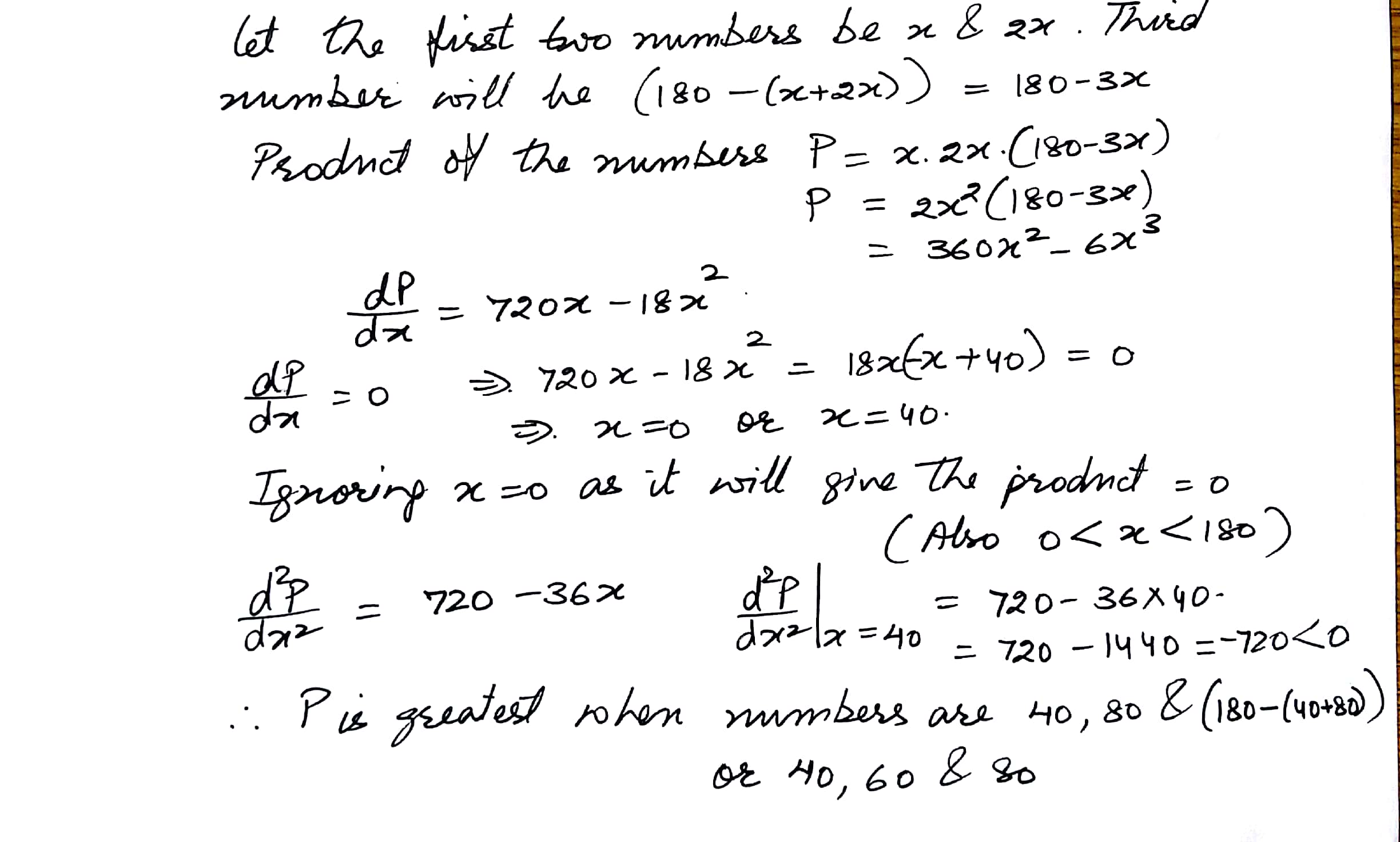5775

Mathematics Integration Level: Misc Level

Three numbers are given whose sum is 180 and the ratio of first two of them is 1:2 if the product of the numbers is greatest, find the numbers